## Fluids

Topics relate to Fluent, CFX, Turbogrid and more

•xing
Subscriber

We are trying to simulate flow above 15 triangular bedforms (triangular shapes at the base called dunes) in the water bed.

# 1. Open channel flow model (Inlet boundary: Pressure Inlet with velocity specification. Outlet: Pressure Outlet) – Problem: The inlet velocity value decreases (doesn’t remain same as specified at the inlet) after running simulation for a while.

#2. Non-Open Channel Flow method i.e., by specifying the regions for air and water volume fractions and then patching them (Inlet boundary: velocity inlet. Outlet: Pressure outlet with hydrostatic pressure profile specified for the water) -  Problem: Velocity value remains as specified but there is a jump in the free surface right at the inlet boundary.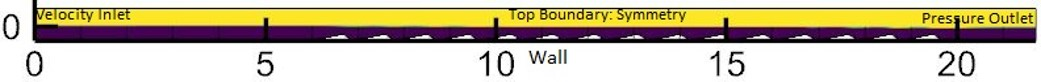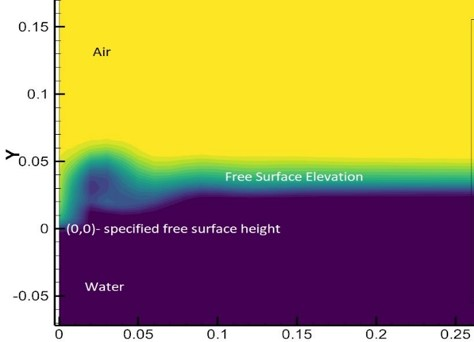We don't have the free surface jump problems using a rectangular domain without triangle shapes at the water bed.

•Rob
Ansys Employee

Open channel VOF should deal with this nicely, and I'd use a velocity inlet. Maybe make the domain a bit higher if the air is being accelerated over the dunes?

•xing
Subscriber

Open Channel Flow model only allows Pressure inlet or Mass Flow Rate Inlet boundary condition. There is an option for specifying ‘velocity’ within Pressure inlet for open channel flow model but this velocity decreased after some time of calculation (only for this dune case for some reason). After searching the Fluent manual we found, “For pressure inlet boundaries, input for Velocity Magnitude is required to calculate the dynamic pressure being used in the total pressure calculation. Note: -The provided Velocity Magnitude is not applied to the boundary.”

Therefore, we tried “Open Channel flow with wave boundary condition” model for the same simulation. This eliminated the velocity reduction problem that was there for the Open channel flow model without wave BC but we have a free surface jump near the inlet. Is this method correct? Is that jump physical? If not, would you have any suggestion to resolve?

•Konstantin
Ansys Employee
Hello,

Could you please try using the Mass Flow Rate at an inlet for the Open Channel Flow condition without wave boundary condition?

The mass flow rate for each phase associated with the flow id defined by:

m_phase_dot = rho_phase*(Area_phase)*(Velocity)

where Area_phase should be readily available based on the height of the interface and the width of the channel at the inlet.

This should help preseve the velocity as well.

Thanks
•xing
Subscriber

Hello:

We checked the mass flow inlet BC. Velocity makes sense (same as we specified) and there is no jump in the free surface (like we had for previous case, kind of wave breaking right at the inlet) BUT the water depth at inlet changed. We specified the free surface to be at Y = 0 but here, the free surface has risen up at all location. I have attached snapshot the three location below.  Also in order to account for the slope in the experiment, we project gravity in vertical and horizontal direction (the angle is small though). Any suggestions?

free surface at inlet: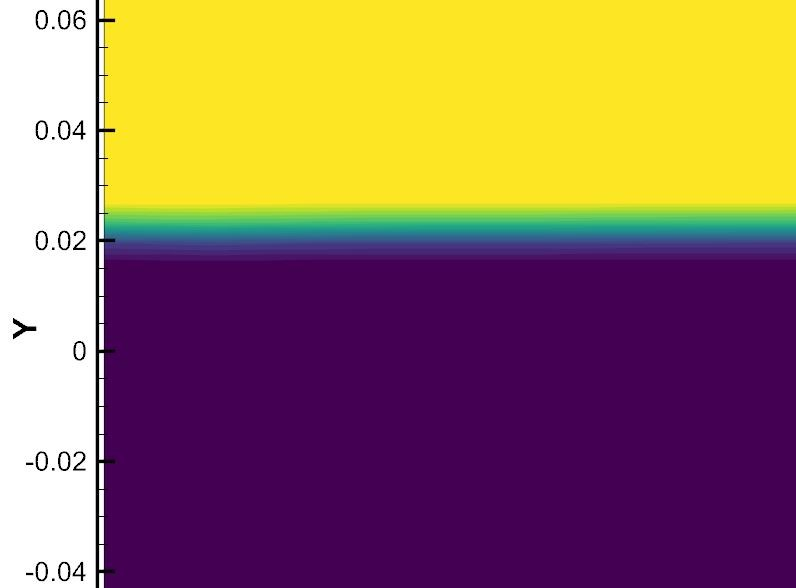free surface above test bedformFree surface at outlet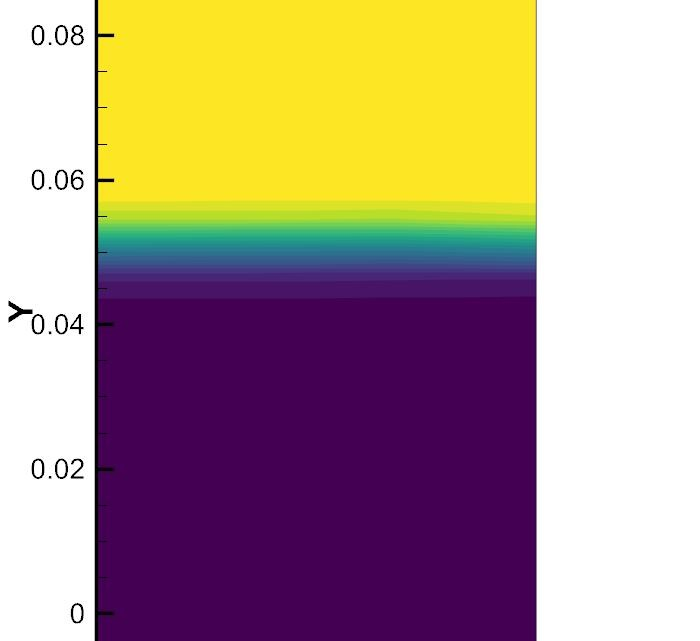•Konstantin
Ansys Employee

Is there a possibility that the presence of the sloped triangles on the bed, actually reduces the effective height (specified at inlet)? So in order to maintain the prescribed mass flow rate, the overall height of water increases. It is also not very clear about the specification of the gravity as you have mentioned. If you have the slopes in the model, then why do you need to modify gravity?

Maybe I am missing something here.

•xing
Subscriber

Dear kkourbat, the experiment has a slope (inlet is slightly higher than the outlet). Since we are using a horizontal and vertical planar domain, we projected the gravity force into both vertical and horizontal directions as shown below. You do have a good point here.  The experiments were performed in three dimensional (3D) whereas our simulations are performed at 2D.  What is the default depth (normal to the page) FLUENT assumes, is it 1m as described here (https://www.cfd-online.com/Forums/fluent/187404-mass-flow-inlet-profile-3d-2d.html), what is the right way to specify the RIGHT flow rate at inlet in 2D CFD when we know the flow rate at 3D in experiments?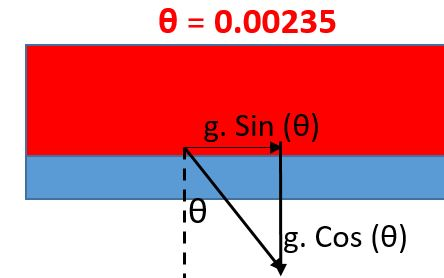•Rob
Ansys Employee

I'd use work out the case to get the same inlet velocity.

Regarding the free surface position, what does the result look like with node values off?  Ie is the change correct or an artefact of the mesh.

•ydai
Ansys Employee

if inflow water mass flow rate through your 3D channel inlet is calculated with a inlet water velocity Vin, water phase inflow area Ain,w = H * W, where H is the water height and W is the channel width, and together with the water phase density Rho_w,  the equivalent 2D water volume rate Vol2D,w should be set as the one that has the same inlet water velocity Vin as in 3D channel, but with the 2D channel width as being W2D = 1m, so Vol2D = Vin * H * W2D. Therefore, the mass rate for the 2D channel flow is, Mrate2D  = Rho_w * Vol2D.

The water level rising in the entrance channel section is perhaps due to the transient mass accumulation of the water phase at the initial stage of the simulation. Water level will be kept as constant height when the mass inflow and outflow at any section of the channel will be equal.

Is it true that your flow domain is consists of 3 section, flat inflow section, and outflow section, and the triangle dune section in the middle between the inflow and outflow sections? It is expected if the answer is yes. If the answer is no, and the inflow water enters the dune as soon as entering the channel, the water wave would be possibly induced by the dune, and the water wave would be generated on the water surface. The wave on the surface will propagate in the speed Uwave = square (g * H) relative to the surface in both directions, towards downstream and upper stream. When the inflow water velocity Vin is higher than Uwave, the wave will not affect the inflow condition, however when Vin < Uwave, inflow condition will be affected by the wave. For more details, please refer to the user's guide one open channel flow.